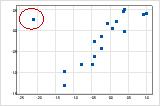# Interpret the key results for Scatterplot

Complete the following steps to interpret a scatterplot.

## Step 1: Look for a model relationship and assess its strength

Determine which model relationship best fits your data and assess the strength of the relationship. If a model fits well, you can use the regression equation for that model to describe your data.

###### Tip

To see how well a particular model fits your data, add a fitted regression line. Double-click the graph. With the graph in editing mode, right-click the graph, then choose Add > Regression Fit. You can hold the pointer over the fitted regression line to see the regression equation.

### Type of relationship

Determine which model relationship, if any, best fits your data. The following are examples of the types of relationships you can model with a regression fit line.

If your data seem to fit a model, you can explore the relationship using a regression analysis.

### Strength of relationship

Assess how closely the data fit the model to estimate the strength of the relationship between X and Y. When the relationship is strong, the regression equation models the data accurately. If you have a fitted regression line, hold the pointer over it to view the regression equation and the R-squared value. The higher the R-squared value, the more accurately the regression equation models your data.

To quantify the strength of a linear (straight) relationship, use a correlation analysis.

## Step 3: Look for other patterns

Outliers may indicate unusual conditions in your data. Time-based trends may indicate changing data conditions.

### Outliers

Outliers, which are data values that are far away from other data values, can strongly affect your results.

On a scatterplot, isolated points identify outliers.Try to identify the cause of any outliers. Correct any data entry or measurement errors. Consider removing data values that are associated with abnormal, one-time events (special causes). Then, repeat the analysis.

By using this site you agree to the use of cookies for analytics and personalized content.  Read our policy